# dials.estimate_resolution¶

## Introduction¶

Estimate a resolution limit based on merging statistics calculated in resolution bins.

A number of metrics are supported for estimating a resolution limit, including:

• cc_half (this is the default)

• isigma (unmerged <I/sigI>)

• misigma (merged <I/sigI>)

• i_mean_over_sigma_mean (unmerged <I>/<sigI>)

• cc_ref (CC vs provided reference data set)

• completeness

• rmerge

Resolution estimation is performed by fitting an appropriate curve to the relevant merging statistics calculated in resolution bins (with a roughly equal number of reflections per bin). The estimated resolution limit is chosen as the resolution at which the fitted function equals the specified criteria.

If multiple metrics are requested, the chosen resolution limit will be the lowest resolution value estimated across the selected metrics.

The fitting functions for the various metrics are defined as follows:

• cc_half: fit a tanh function the form (1/2)(1 - tanh(z)) where z = (s - s0)/r, s0 is the value of s at the half-falloff value, and r controls the steepness of falloff

• isigma, misigma, i_mean_over_sigma_mean: fit a polynomial to the values log(y(x))

• rmerge: fit a polynomial to the values log(1/y(x))

• completeness: fit a polynomial to the values y(x)

## Example use cases¶

Run with defaults on scaled data:

```dials.estimate_resolution scaled.expt scaled.refl
```

Run with default on scaled unmerged mtz file:

```dials.estimate_resolution scaled_unmerged.mtz
```

Override the default cc_half cutoff:

```dials.estimate_resolution scaled.expt scaled.refl cc_half=0.1
```

Use merged <I/sigI> resolution cutoff instead of cc_half:

```dials.estimate_resolution scaled.expt scaled.refl misigma=1.0 cc_half=None
```

Use unmerged <I/sigI> resolution cutoff in addition to default cc_half:

```dials.estimate_resolution scaled.expt scaled.refl isigma=0.25
```

Use cc_ref resolution cutoff:

```dials.estimate_resolution cc_ref=0.3 cc_half=None reference=reference.mtz
```

Example curve fits and resulting resolution estimates using various metrics: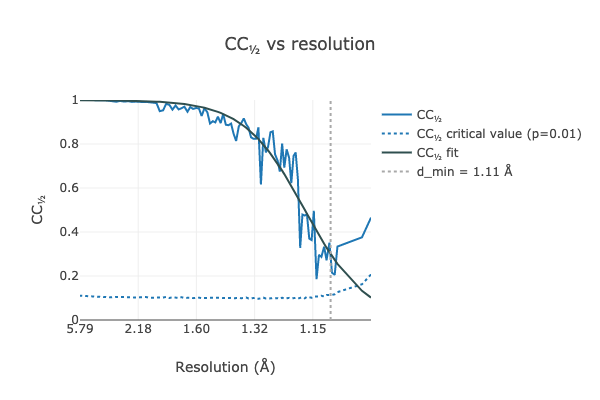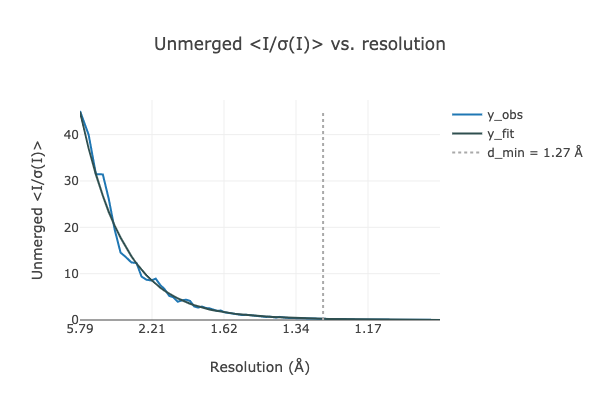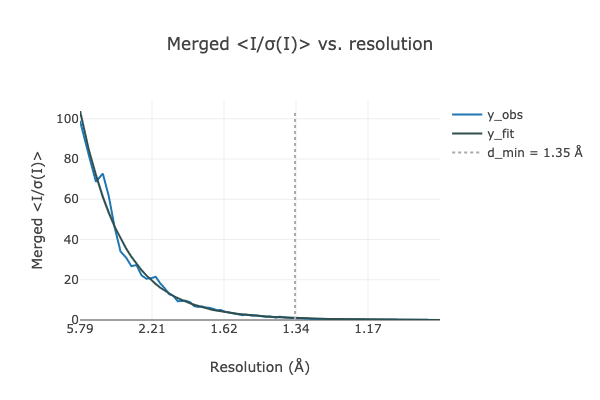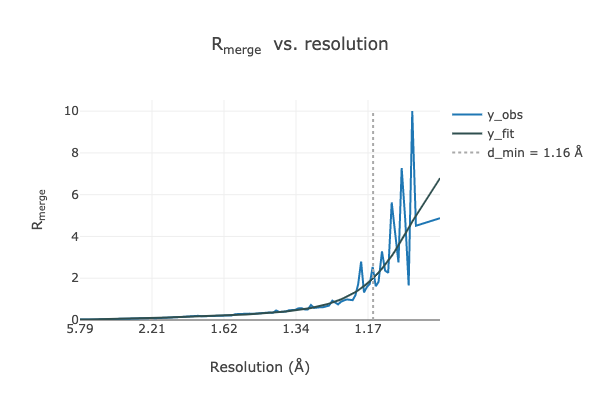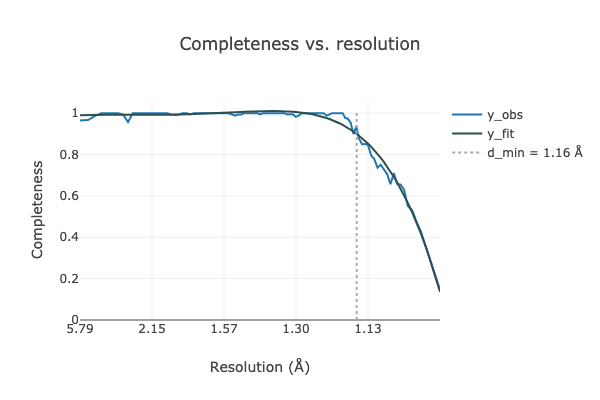## Basic parameters¶

```resolution {
cc_half_method = *half_dataset sigma_tau
reflections_per_bin = 10
labels = None
reference = None
emax = 4
batch_range = None
}
output {
log = dials.estimate_resolution.log
html = dials.estimate_resolution.html
json = None
}
```

## Full parameter definitions¶

```resolution {
rmerge = None
.help = "Maximum value of Rmerge in the outer resolution shell"
.short_caption = "Outer shell Rmerge"
.type = float(value_min=0, allow_none=True)
.expert_level = 1
completeness = None
.help = "Minimum completeness in the outer resolution shell"
.short_caption = "Outer shell completeness"
.type = float(value_min=0, allow_none=True)
.expert_level = 1
cc_ref = 0.1
.help = "Minimum value of CC vs reference data set in the outer resolution"
"shell"
.short_caption = "Outer shell CCref"
.type = float(value_min=0, allow_none=True)
.expert_level = 1
cc_half = 0.3
.help = "Minimum value of CC½ in the outer resolution shell"
.short_caption = "Outer shell CC½"
.type = float(value_min=0, allow_none=True)
.expert_level = 1
cc_half_method = *half_dataset sigma_tau
.type = choice
cc_half_significance_level = 0.1
.type = float(value_min=0, value_max=1, allow_none=True)
.expert_level = 1
cc_half_fit = polynomial *tanh
.type = choice
.expert_level = 1
isigma = None
.help = "Minimum value of the unmerged <I/sigI> in the outer resolution"
"shell"
.short_caption = "Outer shell unmerged <I/sigI>"
.type = float(value_min=0, allow_none=True)
.expert_level = 1
misigma = None
.help = "Minimum value of the merged <I/sigI> in the outer resolution"
"shell"
.short_caption = "Outer shell merged <I/sigI>"
.type = float(value_min=0, allow_none=True)
.expert_level = 1
i_mean_over_sigma_mean = None
.help = "Minimum value of the unmerged <I>/<sigI> in the outer resolution"
"shell"
.short_caption = "Outer shell unmerged <I>/<sigI>"
.type = float(value_min=0, allow_none=True)
.expert_level = 2
nbins = 100
.help = "Maximum number of resolution bins to use for estimation of"
"resolution limit."
.short_caption = "Number of resolution bins."
.type = int(allow_none=True)
.expert_level = 1
reflections_per_bin = 10
.help = "Minimum number of reflections per bin."
.type = int(allow_none=True)
binning_method = *counting_sorted volume
.help = "Use equal-volume bins or bins with approximately equal numbers of"
"reflections per bin."
.short_caption = "Equal-volume or equal #ref binning."
.type = choice
.expert_level = 1
anomalous = False
.short_caption = "Keep anomalous pairs separate in merging statistics"
.type = bool
.expert_level = 1
labels = None
.type = strings
space_group = None
.type = space_group
.expert_level = 1
reference = None
.type = path
emax = 4
.help = "Reject reflecitons with normalised intensities E^2 > emax^2"
.type = float(value_min=0, allow_none=True)
batch_range = None
.type = ints(size=2, value_min=0)
}
output {
log = dials.estimate_resolution.log
.type = path
html = dials.estimate_resolution.html
.type = path
json = None
.type = path
}
```